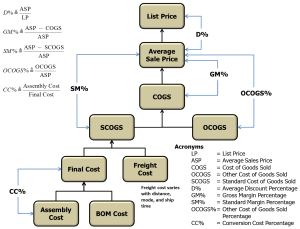# Computing List Price from BOM Cost

Quote of the Day

Not everyone can be a great chef, but a great chef can come from anywhere.

— Ratatouille. This quote is true for every profession.

## IntroductionFigure 1: Relationship of Various Product Costs.
I know its too busy, but this "cheat sheet" helps
me keep all the terms straight in my head.

All engineers must be familiar with the basics of product costing. In this post, I will cover a common product cost model (Figure 1) and provide two examples that illustrate how to calculate the list price of a product given the product's component costs plus some corporate parameters, like average discount rate and required gross margin.

All product cost models are related, but they differ in the details. The model shown in Figure 1 is a common one, but I certainly have used others. This particular model is similar to that used by my son's firm.

The key lesson of this exercise is to show that every dollar spent on components ends up contributing multiple dollars to the list price. This is why I focus so intensely on ensuring that every component pulls its weight in terms of delivering value to customers. To my engineers, I often quote Andrew Carnegie, who is famous for saying "Control costs and profits will follow."

For financial work, I usually use Excel. However, this exercise did involve solving a system of equations, so I used Mathcad. I then used my Mathcad results to check an Excel spreadsheet I cooked up for general use (available here).

## Background

### Definitions of Cost Elements

Bill of Materials (BOM) Cost (symbol CBOM)
The cost of the individual components that make up an assembly. This cost is driven by the product requirements and the implementation approach used by the design engineers. In general, standard component costs are assumed – standard costs are a baseline set for each component each quarter. Any component cost variances from the standard are accounted for in OCOGS.
Assembly Cost (symbol CAsm)
The cost of assembling the components into a product. This cost does not include the freight charge associated with shipping the product from the manufacturing site to a distribution center.
Final Cost (symbol CFinal)
This is a non-standard nomenclature. It is simply the sum of the BOM cost and assembly cost.
Freight Cost (symbol CFreight)
Cost of shipping the product to a distribution center. This cost is highly variable because it depends on how you package the product (e.g. one per box, multiple per box), the distance shipped, and the mode of transportation (e.g. airplane versus container ship).
Standard Cost of Goods Sold (symbol SCOGS)
The sum of the final cost and freight.
Other Cost of Goods Sold (symbol OCOGS)
Sometimes issues arise in manufacturing a product that results in variances in our BOM cost from the standard. These variances include things like purchase part variances (i.e. unexpected part cost changes from the standard), excess and obsolete charges, and expedite fees.
Cost of Goods Sold (symbol COGS)
COGS is the cost of creating the products that a company sells – it only includes those costs that are directly tied to the production of the product.
Average Sales Price (ASP)
The average price for which the product was sold. This number is important because it is used to compute revenue R, i.e.$R=ASP\cdot N$, where N is the number of units sold.
List Price (symbol LP)
The advertised price of a product.

### Definitions of Corporate Parameters

Gross Margin Percentage (symbol GM%)
The percentage of profit in the ASP. GM% relates COGS to ASP using the equation$COGS=\left( {1-GM\%} \right)\cdot ASP$.
OCOGS Percentage (symbol OCOGS%is)
The percentage of OCOGS in the ASP. OCOGS% relates OCOGS to ASP using the equation$OCOGS=OCOGS\%\cdot ASP$.
Conversion Cost Percentage (symbol CC%)
The assembly cost percentage in the final cost. Because freight varies with distance and mode of shipment, CC% never includes freight cost to ensure that assembly rates can be directly compared. CC% is used to compute assembly cost using the equation${{C}_{{Asm}}}=\frac{{CC\%}}{{1-CC\%}}\cdot {{C}_{{BOM}}}$.
Discount (symbol D%)
The average discount received by customers. Discount is used to relate LP to ASP through the equation$ASP=\left( {1-D\%} \right)\cdot LP$.

My plan here is to show how to compute the list price of a mythical product with a total component cost of $100. All the corporate parameters I will be assuming are similarly mythical. ## Analysis ### Approach With the exception of OCOGS – which is a function of SCOGS, OCOGS%, and GM% – all the equations are straightforward and given in the definitions above. I derive an equation for OCOGS in Figure 2. ### Example: Compute List Price Given BOM Cost Figure 3 show how you can compute the list price of a product given the BOM cost, freight cost, and the corporate parameters. ### Example: Compute BOM Cost Given List Price Figure 4 show how you can compute the BOM cost of a product given the list price, freight cost, and the corporate parameters. This example is the reverse of the example in Figure 3. ## Conclusion In this example, a$1 of BOM cost converts to nearly $3 of list price. This is not an unusual ratio. For example, when I worked at HP in the 80's,$1 of BOM cost converted to \$4 of list price.

I have wanted to prepare a costing/pricing calculation example for some time – I finally got around to it. The math is not difficult – you just need to keep things organized.

This entry was posted in Financial. Bookmark the permalink.